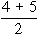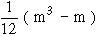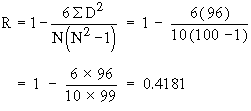Support the Monkey! Tell All your Friends and TeachersHome MonkeyNotes Printable Notes Digital Library Study Guides Study Smart Parents Tips College Planning Test Prep Fun Zone Help / FAQ How to Cite Request a New Title

1. Find the difference D = R1 - R2
where R1 = Rank of x and R2 = Rank of y
Note that
S D = 0 (always)

2. Calculate D2 and then find S D2

3. Apply the formula.

¬ Note :

In some cases, there is a tie between two or more items. in such a case each items have ranks 4th and 5th respectively then they are given= 4.5th rank. If three items are of equal rank say 4th then they are given= 5th rank each. If m be the number of items of equal ranks, the factoris added to S D2. If there are more than one of such cases then this factor added as many times as the number of such cases, thenExample Calculate  R  from the following data.

 Student No.: 1 2 3 4 5 6 7 8 9 10 Rank in Maths : 1 3 7 5 4 6 2 10 9 8 Rank in Stats: 3 1 4 5 6 9 7 8 10 2Your browser does not support the IFRAME tag.
Solution :

 Student No. Rank in Maths (R1) Rank in Stats (R2) R1 - R2 D (R1 - R2 )2 D2 1 1 3 -2 4 2 3 1 2 4 3 7 4 3 9 4 5 5 0 0 5 4 6 -2 4 6 6 9 -3 9 7 2 7 -5 25 8 10 8 2 4 9 9 10 -1 1 10 8 2 6 36 N = 10 S D = 0 S D2 = 96

Calculation of R :Index

6. 1 Introduction
6. 2 Correlation
6. 3 Types of Correlation
6. 4 Degrees of Correlation
6. 5 Methods of determining correlation
6. 6 Coefficients of Correlation for Bivariate Grouped Data
6. 7 Probable Error
6. 8 Rank Correlation Coefficient
6. 9 Linear Regression

Chapter 7Search: All Products Books Popular Music Classical Music Video DVD Toys & Games Electronics Software Tools & Hardware Outdoor Living Kitchen & Housewares Camera & Photo Cell Phones Keywords: